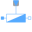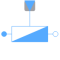# Information

This information is part of the Modelica Standard Library maintained by the Modelica Association.

The admittance model represents a parallel connection of a resistor and either a capacitor or inductor in each phase.The linear admittance connects the complex voltage `v` with the complex current `i` by `v*Y = i` in each phase, using `m` variable singlephase admittances. The admittances `Y_ref = G_ref + j*B_ref` are given as complex input signals, representing the resistive and reactive components of the input admittances. The resistive components are modeled temperature dependent, so the real part `G_actual = real(Y)` are determined from the actual operating temperatures and the reference input conductances `real(Y_ref)`. Conditional heat ports are considered. The reactive components `B_actual = imag(Y)` are equal to `imag(Y_ref)` if `frequencyDependent = false`. Frequency dependency is considered by `frequencyDependent = true`, distinguishing two cases:

(a) `imag(Y_ref) > 0`: capacitive case
The actual susceptances `B_actual` are proportional to `f/f_ref`
(b) `imag(Y_ref) < 0`: inductive case
The actual susceptances `B_actual` are proportional to `f_ref/f`

#### Note

Zero crossings of the real or imaginary parts of the admittance signals `Y_ref` could cause singularities due to the actual structure of the connected network.

plug_p plug_n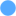Type: PositivePlug Description: Positive quasi-static polyphase plug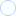Type: NegativePlug Description: Negative quasi-static polyphase plug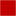Type: HeatPort_a[mh] Description: Conditional heat ports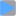Type: ComplexInput[m] Description: Variable complex admittances
v i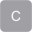Type: ComplexVoltage[m] Description: Complex voltageType: ComplexCurrent[m] Description: Complex current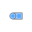Type: PlugToPins_p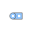Type: PlugToPins_n